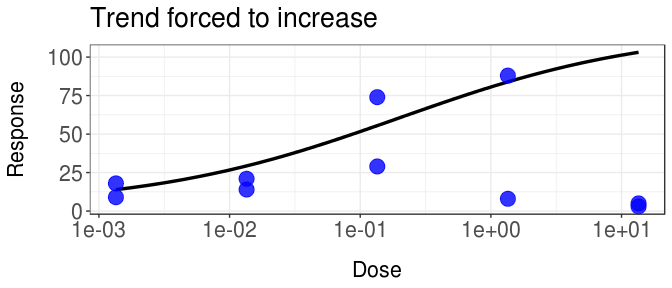# R package dr4pl usage examples

#Installing packages

library(drc)
library(dr4pl)
library(ggplot2)
library(matrixcalc)

#error cases

dr4pl is a four parameter regression tool that was designed to provide a converging function in the pressense of outliers. Consider the next four data sets provided by dr4pl.

Lets first consider the data set drc_error_1.

ggplot(drc_error_1, aes(x = Dose, y = Response)) +
geom_point() +
scale_x_log10() +
ggtitle("drc_error_1")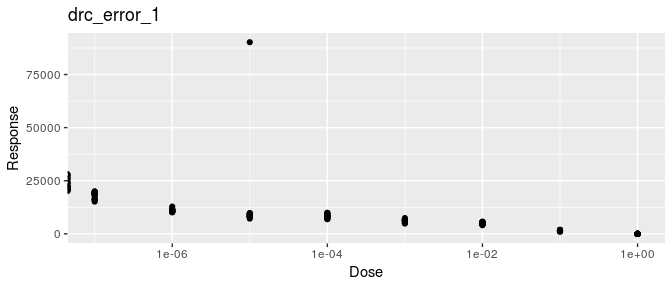As you can see, this data set contains an extreme outlier at one of the dosage levels. These outliers may be a common phenomena with measuring mistakes of some lab instruments. If you were to try and produce a four parameter logistic model with drc, you would recieve the following.

a <- tryCatch({
drc::drm(Response~Dose, data = drc_error_1, fct = LL.4())
},
warning = function(war) {
# warning handler picks up where error was generated
print(paste(war))
},
error = function(err) {
# error handler picks up where error was generated
print(paste(err))
})
##  "simpleWarning in log(dose/parmMat[, 4]): NaNs produced\n"

Instead of removing the extreme outlier, you may try to use dr4pl instead.

a <-tryCatch({
dr4pl(Response~Dose, data = drc_error_1, method.init = "logistic", method.robust = "Tukey")
},
warning = function(war) {
# warning handler picks up where error was generated
print(paste(war))
},
error = function(err) {
# error handler picks up where error was generated
print(paste(err))
})
plot(a, text.title = "Error plot #1")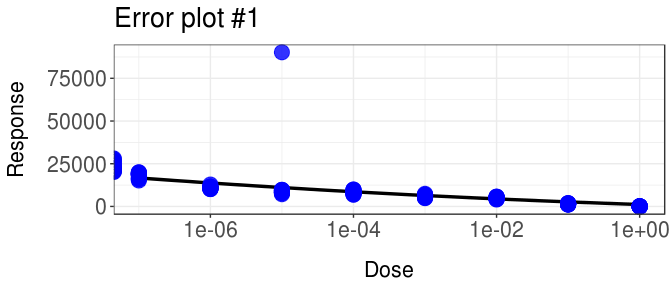Lets next consider the next data set, drc_error_2.

ggplot(drc_error_2, aes(x = Dose, y = Response)) +
geom_point() +
scale_x_log10() +
ggtitle("drc_error_2")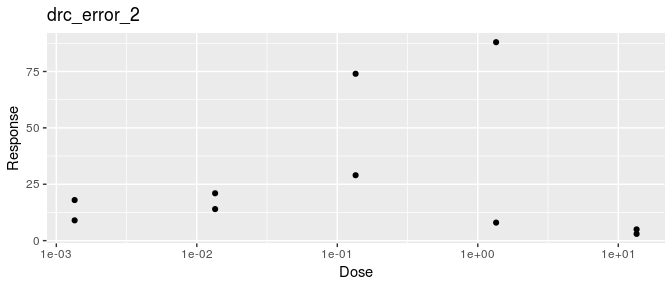This data set suffers from a low sample amount. There are also high outliers at two seperate dose levels. Lets try to plot this case with drc.

a <- tryCatch({
drc::drm(Response~Dose, data = drc_error_2, fct = LL.4())
},
warning = function(war) {
# warning handler picks up where error was generated
print(paste(war))
},
error = function(err) {
# error handler picks up where error was generated
print(paste(err))
})
##  "simpleWarning in log(dose/parmMat[, 4]): NaNs produced\n"

Lets see what we can plot with dr4pl.

a <-tryCatch({
dr4pl(Response~Dose, data = drc_error_2, trend = "decreasing", method.optim = "CG")
},
warning = function(war) {
# warning handler picks up where error was generated
print(paste(war))
},
error = function(err) {
# error handler picks up where error was generated
print(paste(err))
})
b <- plot(a, breaks.x = c(0.00135, 0.0135, 0.135, 1.35, 13.5), text.title = "Error plot #2")
b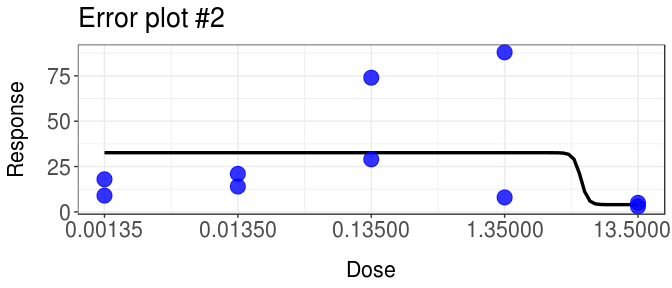Lets next consider the next data set, drc_error_3.

ggplot(drc_error_3, aes(x = Dose, y = Response)) +
geom_point() +
scale_x_log10() +
ggtitle("drc_error_3")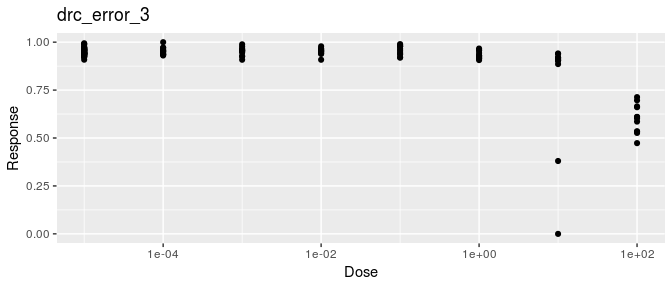This data set presents multiple outliers at one dosage level. Again these outliers may manifest as measurement issues with lab instruments. Additionally this data set exemplifies the problem we refer to as the support problem. The support problem occurs when there is lack of data at either the right or left of the IC50 parameter. Lets try to use drc on this data set.

a <- tryCatch({
drc::drm(Response~Dose, data = drc_error_3, fct = LL.4())
},
warning = function(war) {
# warning handler picks up where error was generated
print(paste(war))
},
error = function(err) {
# error handler picks up where error was generated
print(paste(err))
})
##  "simpleWarning in log(dose/parmMat[, 4]): NaNs produced\n"

Now lets use dr4pl.

a <-tryCatch({
dr4pl(Response~Dose, data = drc_error_3, method.init = "Mead", method.robust = "Huber")
},
warning = function(war) {
# warning handler picks up where error was generated
print(paste(war))
},
error = function(err) {
# error handler picks up where error was generated
print(paste(err))
})
plot(a, text.title = "Error plot #3")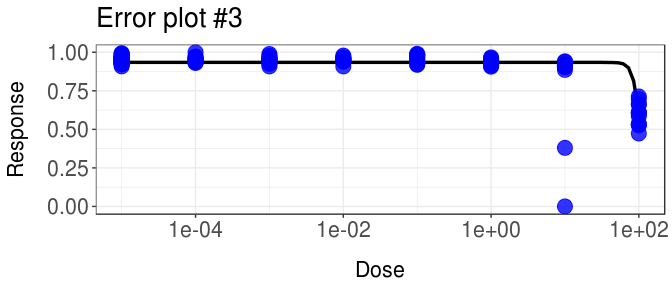Lets next consider the next data set, drc_error_4.

ggplot(drc_error_4, aes(x = Dose, y = Response)) +
geom_point() +
scale_x_log10() +
ggtitle("drc_error_4")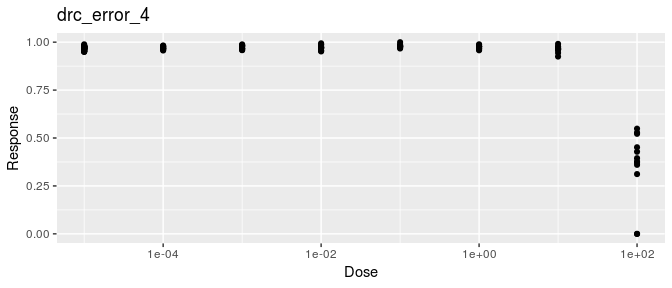This data set has two outliers in the largest dosage level. This data set also exemplifies the support problem as well. Lets try to apply drc to this data set.

a <- tryCatch({
drc::drm(Response~Dose, data = drc_error_4, fct = LL.4())
},
warning = function(war) {
# warning handler picks up where error was generated
print(paste(war))
},
error = function(err) {
# error handler picks up where error was generated
print(paste(err))
})
##  "simpleWarning in log(dose/parmMat[, 4]): NaNs produced\n"

Now lets use dr4pl.

a <-tryCatch({
dr4pl(Response~Dose, data = drc_error_4, method.init = "logistic")
},
warning = function(war) {
# warning handler picks up where error was generated
print(paste(war))
},
error = function(err) {
# error handler picks up where error was generated
print(paste(err))
})
plot(a, text.title = "Error plot #4")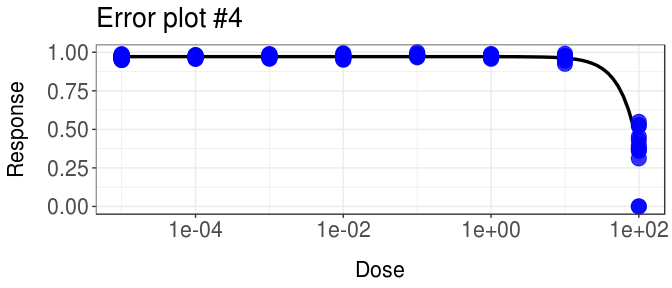The package drc draws errors with each one of these cases. However dr4pl is able generate a curve despite the outliers in each error case. In each case we were able to modify the title and axis names with text.title, text.x, or text.y. We are also able to bring attention to outlier points by passing a vector of the indices to the indices.outlier argument. As seen the the second error case, we may change the x-axis’ and y-axis’ break points by using breaks.x and breaks.y respectively.

#General Usage

dr4pl provides several methods and loss functions for the user to obtain the best fit possible. Lets explore some of the possibities.

Consider you want to plot the dr4pl data set sample_data_5. Lets try and apply the default parameters.

a <- dr4pl(Response~Dose, data = sample_data_5)
plot(a, text.title = "Default Sample data #5")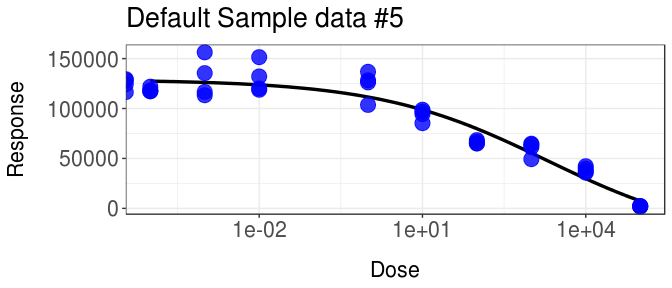After producing a plot with the default parameters, you may be confident in producing a better fit with other parameters. This is where the arguments method.init and method.robust come into play, which you may have noticed that these arguments where used in the error case examples. The default paramter to method.init is “Mead”. This uses the Mead’s method when approximating the theta parameters. The alternative to using Mead’s method is “logistic”, which uses a logistic method to approximate the theta parameters. Lets see how our plot will change when we use the logistic method.

a <- dr4pl(Response~Dose, data = sample_data_5, method.init = "logistic")
plot(a, text.title = "Logistic Method")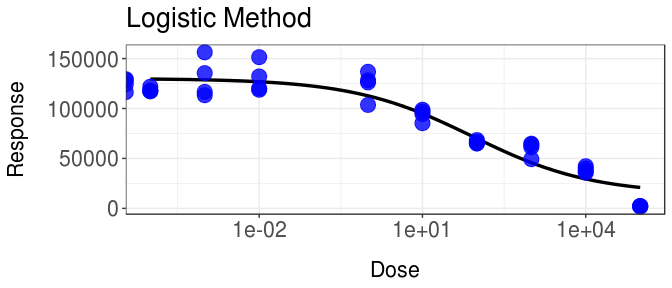Mead’s method seems to generate tighter fit with this data set. Now lets talk about method.robust. method.robust allows the user to choose the loss function used during optimization. The default loss function used is the squared loss function. The user has three other loss functions to select from: “absolute”, “Huber”, and “Tukey”. Lets see how this plot will change when using “absolute” versus “Tukey”.

a <- dr4pl(Response~Dose, data = sample_data_5, method.robust = "absolute")
plot(a, text.title = "Absolute loss function")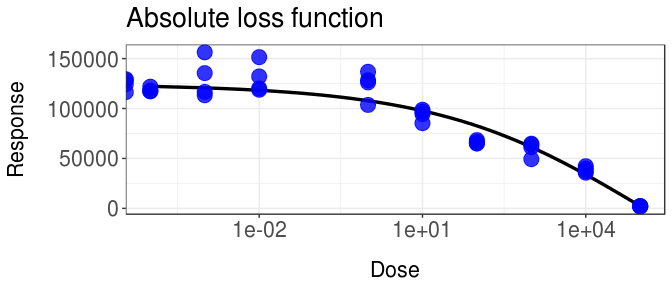a <- dr4pl(Response~Dose, data = sample_data_5, method.init = "Mead", method.robust = "Tukey")
plot(a, text.title = "Mead's method & Tukey's biweight")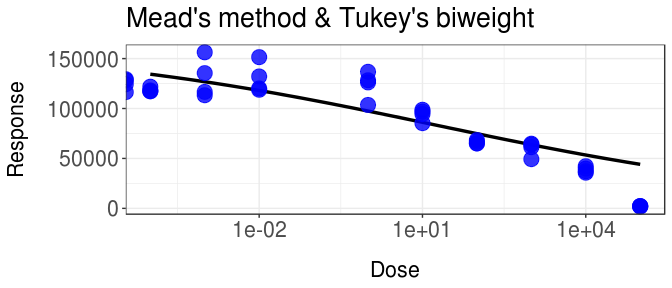When selecting “Tukey” for the argument method.robust, the generated fit is less weighted by the largest dosage level which is an outlier response of zero. When selecting “absolute” for the argument method.robust, the generated fit is more weignted by the largest dosage level. It may take several attempts to find the best parameters for each data set.

Lets look at other uses for this code. Consider the following data set sample_data_3.

a <- dr4pl(Response~Dose, data = sample_data_3, method.init = "Mead")
b <- plot(a, text.title = "Sample data #3")
b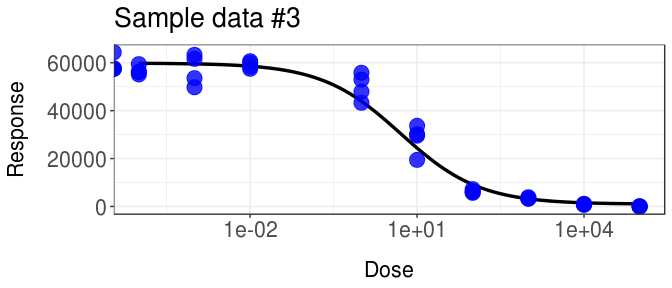Once you produce a curve you feel is representative of your data, you may get the parameters of the curve by using the summary function on the dr4pl variable.

b <- summary(a)
b\$coefficients
##                 Estimate       StdErr         2.5 %        97.5 %
## UpperLimit 59858.0700194 1.328219e-01 58678.1496415 61037.9903974
## IC50           5.0794918 1.531608e-05     3.7133017     6.9483278
## Slope         -0.6117371 1.440712e-05    -0.7397224    -0.4837518
## LowerLimit   980.8205686 1.354448e-01  -222.4002527  2184.0413899

It is possible that you are interested in more than just the IC50 variable. Use the IC() function to produce the respective Dose values.

values <- IC(a, c(10, 30, 50, 70, 90))
values
## InhibPercent:10 InhibPercent:30 InhibPercent:50 InhibPercent:70
##     184.3784198      20.2930770       5.0794918       1.2714305
## InhibPercent:90
##       0.1399363

You may then easily edit your plot further with basic ggplot2 additional controls.

You may use dr4pl on increasing and decreasing curves. Lets see how dr4pl handles the drc data set chekcweed0.

a <- dr4pl(count~time, data = chickweed0, trend = "increasing")
plot(a, text.x = "Time", text.y = "Count", text.title = "drc chickweed0 plot", breaks.x = c(25, 100, 175, 250))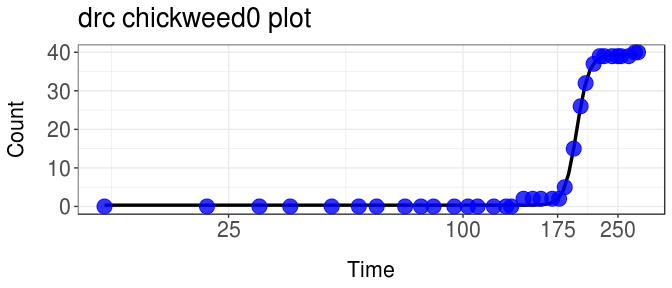You may also force either an increasing or decreasing fit with the parameter trend. trend is best used when the data set is rather ambigious to whether the fit should increase or decrease. dr4pl’s default parameter automizes this and will attempt the best based on the optimization. Let us see how this example plays out with the dr4pl data set drc_error_2.

a <- dr4pl(Response~Dose, data = drc_error_2)
plot(a, text.title = "Trend is default")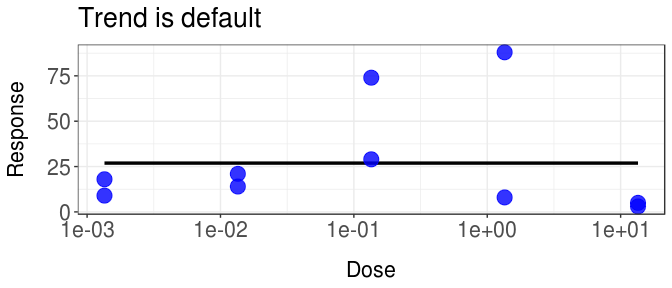If you were to use the summary function, you would see that dr4pl has deemed this plot decreasing, however this plot may not be very satisfying. The parameter trend helps force the fit to either “increasing” or “decreasing” in the event that you are confident that your response follows that trend. We may choose to force a decreasing curve, with other apporpriate parameters.

a <- dr4pl(Response~Dose, data = drc_error_2, trend = "decreasing", method.optim = "CG")
plot(a, text.title = "Trend forced to decrease")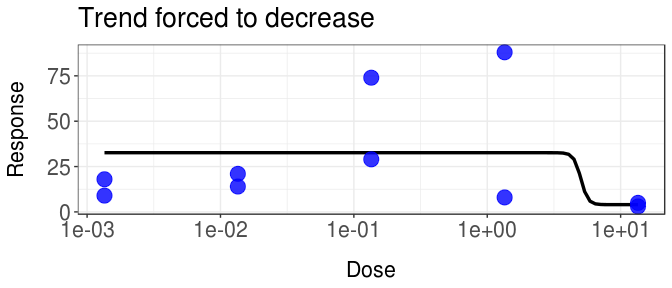We may also choose to force an increasing curve.

a <- dr4pl(Response~Dose, data = drc_error_2, trend = "increasing", method.robust = "Tukey", method.optim = "SANN")
plot(a, text.title = "Trend forced to increase")